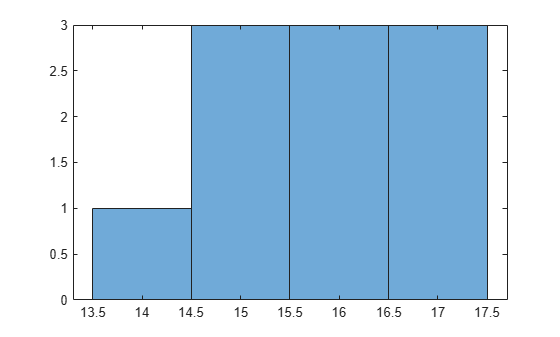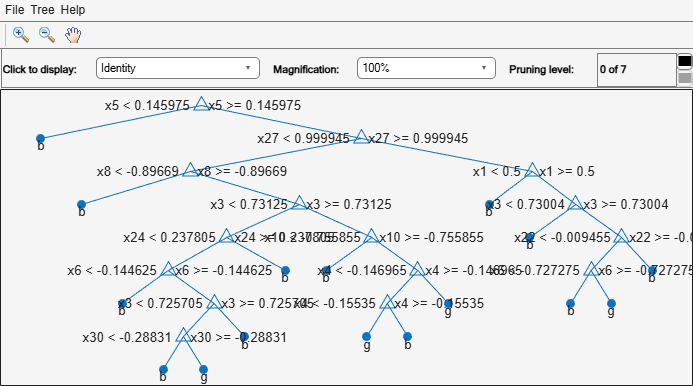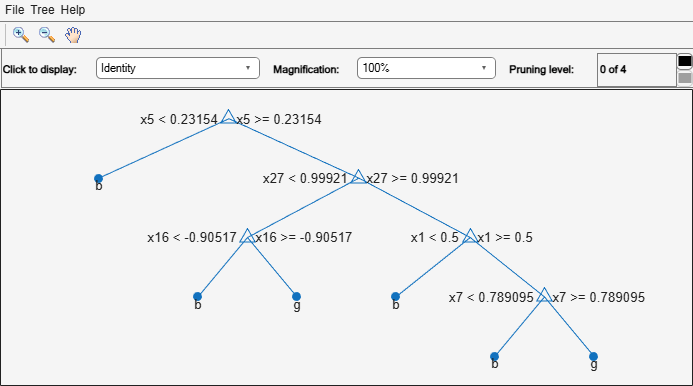# ClassificationTree class

Superclasses: `CompactClassificationTree`

Binary decision tree for multiclass classification

## Description

A `ClassificationTree` object represents a decision tree with binary splits for classification. An object of this class can predict responses for new data using the `predict` method. The object contains the data used for training, so it can also compute resubstitution predictions.

## Construction

Create a `ClassificationTree` object by using `fitctree`.

## Properties

 `BinEdges` Bin edges for numeric predictors, specified as a cell array of p numeric vectors, where p is the number of predictors. Each vector includes the bin edges for a numeric predictor. The element in the cell array for a categorical predictor is empty because the software does not bin categorical predictors.The software bins numeric predictors only if you specify the `'NumBins'` name-value argument as a positive integer scalar when training a model with tree learners. The `BinEdges` property is empty if the `'NumBins'` value is empty (default).You can reproduce the binned predictor data `Xbinned` by using the `BinEdges` property of the trained model `mdl`.```X = mdl.X; % Predictor data Xbinned = zeros(size(X)); edges = mdl.BinEdges; % Find indices of binned predictors. idxNumeric = find(~cellfun(@isempty,edges)); if iscolumn(idxNumeric) idxNumeric = idxNumeric'; end for j = idxNumeric x = X(:,j); % Convert x to array if x is a table. if istable(x) x = table2array(x); end % Group x into bins by using the `discretize` function. xbinned = discretize(x,[-inf; edges{j}; inf]); Xbinned(:,j) = xbinned; end````Xbinned` contains the bin indices, ranging from 1 to the number of bins, for numeric predictors. `Xbinned` values are 0 for categorical predictors. If `X` contains `NaN`s, then the corresponding `Xbinned` values are `NaN`s. `CategoricalPredictors` Categorical predictor indices, specified as a vector of positive integers. `CategoricalPredictors` contains index values indicating that the corresponding predictors are categorical. The index values are between 1 and `p`, where `p` is the number of predictors used to train the model. If none of the predictors are categorical, then this property is empty (`[]`). `CategoricalSplit` An n-by-2 cell array, where `n` is the number of categorical splits in `tree`. Each row in `CategoricalSplit` gives left and right values for a categorical split. For each branch node with categorical split `j` based on a categorical predictor variable `z`, the left child is chosen if `z` is in `CategoricalSplit(j,1)` and the right child is chosen if `z` is in `CategoricalSplit(j,2)`. The splits are in the same order as nodes of the tree. Nodes for these splits can be found by running `cuttype` and selecting `'categorical'` cuts from top to bottom. `Children` An n-by-2 array containing the numbers of the child nodes for each node in `tree`, where n is the number of nodes. Leaf nodes have child node `0`. `ClassCount` An n-by-k array of class counts for the nodes in `tree`, where n is the number of nodes and k is the number of classes. For any node number `i`, the class counts `ClassCount(i,:)` are counts of observations (from the data used in fitting the tree) from each class satisfying the conditions for node `i`. `ClassNames` List of the elements in `Y` with duplicates removed. `ClassNames` can be a categorical array, cell array of character vectors, character array, logical vector, or a numeric vector. `ClassNames` has the same data type as the data in the argument `Y`. (The software treats string arrays as cell arrays of character vectors.) `ClassProbability` An n-by-k array of class probabilities for the nodes in `tree`, where n is the number of nodes and k is the number of classes. For any node number `i`, the class probabilities `ClassProbability(i,:)` are the estimated probabilities for each class for a point satisfying the conditions for node `i`. `Cost` Square matrix, where `Cost(i,j)` is the cost of classifying a point into class `j` if its true class is `i` (the rows correspond to the true class and the columns correspond to the predicted class). The order of the rows and columns of `Cost` corresponds to the order of the classes in `ClassNames`. The number of rows and columns in `Cost` is the number of unique classes in the response. This property is read-only. `CutCategories` An n-by-2 cell array of the categories used at branches in `tree`, where n is the number of nodes. For each branch node `i` based on a categorical predictor variable `X`, the left child is chosen if `X` is among the categories listed in `CutCategories{i,1}`, and the right child is chosen if `X` is among those listed in `CutCategories{i,2}`. Both columns of `CutCategories` are empty for branch nodes based on continuous predictors and for leaf nodes. `CutPoint` contains the cut points for `'continuous'` cuts, and `CutCategories` contains the set of categories. `CutPoint` An n-element vector of the values used as cut points in `tree`, where n is the number of nodes. For each branch node `i` based on a continuous predictor variable `X`, the left child is chosen if `X=CutPoint(i)`. `CutPoint` is `NaN` for branch nodes based on categorical predictors and for leaf nodes. `CutPoint` contains the cut points for `'continuous'` cuts, and `CutCategories` contains the set of categories. `CutType` An n-element cell array indicating the type of cut at each node in `tree`, where n is the number of nodes. For each node `i`, `CutType{i}` is: `'continuous'` — If the cut is defined in the form `X < v` for a variable `X` and cut point `v`.`'categorical'` — If the cut is defined by whether a variable `X` takes a value in a set of categories.`''` — If `i` is a leaf node. `CutPoint` contains the cut points for `'continuous'` cuts, and `CutCategories` contains the set of categories. `CutPredictor` An n-element cell array of the names of the variables used for branching in each node in `tree`, where n is the number of nodes. These variables are sometimes known as cut variables. For leaf nodes, `CutPredictor` contains an empty character vector. `CutPoint` contains the cut points for `'continuous'` cuts, and `CutCategories` contains the set of categories. `CutPredictorIndex` An n-element array of numeric indices for the variables used for branching in each node in `tree`, where n is the number of nodes. For more information, see `CutPredictor`. `ExpandedPredictorNames` Expanded predictor names, stored as a cell array of character vectors. If the model uses encoding for categorical variables, then `ExpandedPredictorNames` includes the names that describe the expanded variables. Otherwise, `ExpandedPredictorNames` is the same as `PredictorNames`. `HyperparameterOptimizationResults` Description of the cross-validation optimization of hyperparameters, stored as a `BayesianOptimization` object or a table of hyperparameters and associated values. Nonempty when the `OptimizeHyperparameters` name-value pair is nonempty at creation. Value depends on the setting of the `HyperparameterOptimizationOptions` name-value pair at creation: `'bayesopt'` (default) — Object of class `BayesianOptimization``'gridsearch'` or `'randomsearch'` — Table of hyperparameters used, observed objective function values (cross-validation loss), and rank of observations from lowest (best) to highest (worst) `IsBranchNode` An n-element logical vector that is `true` for each branch node and `false` for each leaf node of `tree`. `ModelParameters` Parameters used in training `tree`. To display all parameter values, enter `tree.ModelParameters`. To access a particular parameter, use dot notation. `NumObservations` Number of observations in the training data, a numeric scalar. `NumObservations` can be less than the number of rows of input data `X` when there are missing values in `X` or response `Y`. `NodeClass` An n-element cell array with the names of the most probable classes in each node of `tree`, where n is the number of nodes in the tree. Every element of this array is a character vector equal to one of the class names in `ClassNames`. `NodeError` An n-element vector of the errors of the nodes in `tree`, where n is the number of nodes. `NodeError(i)` is the misclassification probability for node `i`. `NodeProbability` An n-element vector of the probabilities of the nodes in `tree`, where n is the number of nodes. The probability of a node is computed as the proportion of observations from the original data that satisfy the conditions for the node. This proportion is adjusted for any prior probabilities assigned to each class. `NodeRisk` An n-element vector of the risk of the nodes in the tree, where n is the number of nodes. The risk for each node is the measure of impurity (Gini index or deviance) for this node weighted by the node probability. If the tree is grown by twoing, the risk for each node is zero. `NodeSize` An n-element vector of the sizes of the nodes in `tree`, where n is the number of nodes. The size of a node is defined as the number of observations from the data used to create the tree that satisfy the conditions for the node. `NumNodes` The number of nodes in `tree`. `Parent` An n-element vector containing the number of the parent node for each node in `tree`, where n is the number of nodes. The parent of the root node is `0`. `PredictorNames` Cell array of character vectors containing the predictor names, in the order which they appear in `X`. `Prior` Numeric vector of prior probabilities for each class. The order of the elements of `Prior` corresponds to the order of the classes in `ClassNames`. The number of elements of `Prior` is the number of unique classes in the response. This property is read-only. `PruneAlpha` Numeric vector with one element per pruning level. If the pruning level ranges from 0 to M, then `PruneAlpha` has M + 1 elements sorted in ascending order. `PruneAlpha(1)` is for pruning level 0 (no pruning), `PruneAlpha(2)` is for pruning level 1, and so on. `PruneList` An n-element numeric vector with the pruning levels in each node of `tree`, where n is the number of nodes. The pruning levels range from 0 (no pruning) to M, where M is the distance between the deepest leaf and the root node. `ResponseName` A character vector that specifies the name of the response variable (`Y`). `RowsUsed` An n-element logical vector indicating which rows of the original predictor data (`X`) were used in fitting. If the software uses all rows of `X`, then `RowsUsed` is an empty array (`[]`). `ScoreTransform` Function handle for transforming predicted classification scores, or character vector representing a built-in transformation function. `none` means no transformation, or `@(x)x`. To change the score transformation function to, for example, `function`, use dot notation. For available functions (see `fitctree`), enter`Mdl.ScoreTransform = 'function';`You can set a function handle for an available function, or a function you define yourself by entering`tree.ScoreTransform = @function;` `SurrogateCutCategories` An n-element cell array of the categories used for surrogate splits in `tree`, where n is the number of nodes in `tree`. For each node `k`, `SurrogateCutCategories{k}` is a cell array. The length of `SurrogateCutCategories{k}` is equal to the number of surrogate predictors found at this node. Every element of `SurrogateCutCategories{k}` is either an empty character vector for a continuous surrogate predictor, or is a two-element cell array with categories for a categorical surrogate predictor. The first element of this two-element cell array lists categories assigned to the left child by this surrogate split, and the second element of this two-element cell array lists categories assigned to the right child by this surrogate split. The order of the surrogate split variables at each node is matched to the order of variables in `SurrogateCutPredictor`. The optimal-split variable at this node does not appear. For nonbranch (leaf) nodes, `SurrogateCutCategories` contains an empty cell. `SurrogateCutFlip` An n-element cell array of the numeric cut assignments used for surrogate splits in `tree`, where n is the number of nodes in `tree`. For each node `k`, `SurrogateCutFlip{k}` is a numeric vector. The length of `SurrogateCutFlip{k}` is equal to the number of surrogate predictors found at this node. Every element of `SurrogateCutFlip{k}` is either zero for a categorical surrogate predictor, or a numeric cut assignment for a continuous surrogate predictor. The numeric cut assignment can be either –1 or +1. For every surrogate split with a numeric cut C based on a continuous predictor variable Z, the left child is chosen if Z

## Object Functions

 `compact` Compact tree `compareHoldout` Compare accuracies of two classification models using new data `crossval` Cross-validated decision tree `cvloss` Classification error by cross validation `edge` Classification edge `gather` Gather properties of Statistics and Machine Learning Toolbox object from GPU `lime` Local interpretable model-agnostic explanations (LIME) `loss` Classification error `margin` Classification margins `nodeVariableRange` Retrieve variable range of decision tree node `partialDependence` Compute partial dependence `plotPartialDependence` Create partial dependence plot (PDP) and individual conditional expectation (ICE) plots `predict` Predict labels using classification tree `predictorImportance` Estimates of predictor importance for classification tree `prune` Produce sequence of classification subtrees by pruning `resubEdge` Classification edge by resubstitution `resubLoss` Classification error by resubstitution `resubMargin` Classification margins by resubstitution `resubPredict` Predict resubstitution labels of classification tree `shapley` Shapley values `surrogateAssociation` Mean predictive measure of association for surrogate splits in classification tree `testckfold` Compare accuracies of two classification models by repeated cross-validation `view` View classification tree

## Copy Semantics

Value. To learn how value classes affect copy operations, see Copying Objects.

## Examples

collapse all

Grow a classification tree using the `ionosphere` data set.

```load ionosphere tc = fitctree(X,Y)```
```tc = ClassificationTree ResponseName: 'Y' CategoricalPredictors: [] ClassNames: {'b' 'g'} ScoreTransform: 'none' NumObservations: 351 Properties, Methods ```

You can control the depth of the trees using the `MaxNumSplits`, `MinLeafSize`, or `MinParentSize` name-value pair parameters. `fitctree` grows deep decision trees by default. You can grow shallower trees to reduce model complexity or computation time.

Load the `ionosphere` data set.

`load ionosphere`

The default values of the tree depth controllers for growing classification trees are:

• `n - 1` for `MaxNumSplits`. `n` is the training sample size.

• `1` for `MinLeafSize`.

• `10` for `MinParentSize`.

These default values tend to grow deep trees for large training sample sizes.

Train a classification tree using the default values for tree depth control. Cross-validate the model by using 10-fold cross-validation.

```rng(1); % For reproducibility MdlDefault = fitctree(X,Y,'CrossVal','on');```

Draw a histogram of the number of imposed splits on the trees. Also, view one of the trees.

```numBranches = @(x)sum(x.IsBranch); mdlDefaultNumSplits = cellfun(numBranches, MdlDefault.Trained); figure; histogram(mdlDefaultNumSplits)````view(MdlDefault.Trained{1},'Mode','graph')`The average number of splits is around 15.

Suppose that you want a classification tree that is not as complex (deep) as the ones trained using the default number of splits. Train another classification tree, but set the maximum number of splits at 7, which is about half the mean number of splits from the default classification tree. Cross-validate the model by using 10-fold cross-validation.

```Mdl7 = fitctree(X,Y,'MaxNumSplits',7,'CrossVal','on'); view(Mdl7.Trained{1},'Mode','graph')```Compare the cross-validation classification errors of the models.

`classErrorDefault = kfoldLoss(MdlDefault)`
```classErrorDefault = 0.1168 ```
`classError7 = kfoldLoss(Mdl7)`
```classError7 = 0.1311 ```

`Mdl7` is much less complex and performs only slightly worse than `MdlDefault`.

expand all

## References

 Breiman, L., J. Friedman, R. Olshen, and C. Stone. Classification and Regression Trees. Boca Raton, FL: CRC Press, 1984.

## Version History

Introduced in R2011a